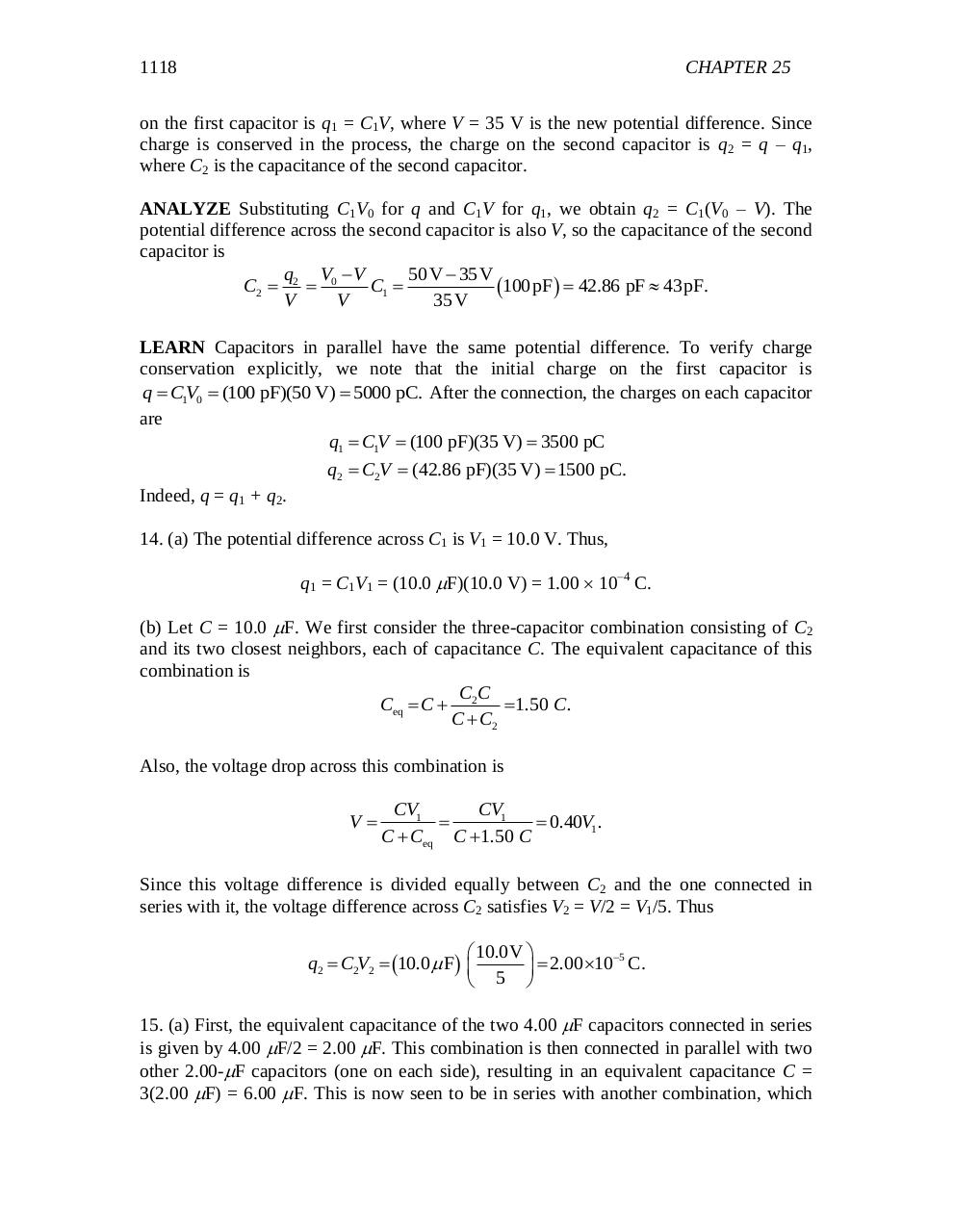# chapter 25.pdfPage 1 2 3 45632

#### Text preview

1118

CHAPTER 25

on the first capacitor is q1 = C1V, where V = 35 V is the new potential difference. Since
charge is conserved in the process, the charge on the second capacitor is q2 = q – q1,
where C2 is the capacitance of the second capacitor.
ANALYZE Substituting C1V0 for q and C1V for q1, we obtain q2 = C1(V0 – V). The
potential difference across the second capacitor is also V, so the capacitance of the second
capacitor is
q V V
50 V  35V
C2  2  0
C1 
100 pF  42.86 pF  43pF.
V
V
35V
LEARN Capacitors in parallel have the same potential difference. To verify charge
conservation explicitly, we note that the initial charge on the first capacitor is
q  C1V0  (100 pF)(50 V)  5000 pC. After the connection, the charges on each capacitor
are
q1  C1V  (100 pF)(35 V)  3500 pC
q2  C2V  (42.86 pF)(35 V)  1500 pC.

Indeed, q = q1 + q2.
14. (a) The potential difference across C1 is V1 = 10.0 V. Thus,
q1 = C1V1 = (10.0 F)(10.0 V) = 1.00  10–4 C.
(b) Let C = 10.0 F. We first consider the three-capacitor combination consisting of C2
and its two closest neighbors, each of capacitance C. The equivalent capacitance of this
combination is
CC
Ceq  C  2  1.50 C.
C  C2
Also, the voltage drop across this combination is

V

CV1
CV1

 0.40V1 .
C  Ceq C  1.50 C

Since this voltage difference is divided equally between C2 and the one connected in
series with it, the voltage difference across C2 satisfies V2 = V/2 = V1/5. Thus
 10.0V 
5
q2  C2V2  10.0  F  
  2.00 10 C.
 5 

15. (a) First, the equivalent capacitance of the two 4.00 F capacitors connected in series
is given by 4.00 F/2 = 2.00 F. This combination is then connected in parallel with two
other 2.00-F capacitors (one on each side), resulting in an equivalent capacitance C =
3(2.00 F) = 6.00 F. This is now seen to be in series with another combination, which